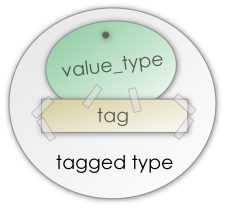#Boost C++ Libraries

...one of the most highly regarded and expertly designed C++ library projects in the world.

### Bimaps with user defined names

In the following example, the library user inserted comments to guide future programmers:

```typedef bimap
<
multiset_of<std::string>,
int

> People;

People people;

// ...

int user_id;
std::cin >> user_id;

// people.right : map<id,name>

People::right_const_iterator id_iter = people.right.find(user_id);
if( id_iter != people.right.end() )
{
// first  : id
// second : name

std::cout << "name: " << id_iter->second << std::endl
<< "id:   " << id_iter->first  << std::endl;
}
else
{
std::cout << "Unknown id, users are:" << std::endl;

// people.left : map<name,id>

for( People::left_const_iterator
name_iter = people.left.begin(),
iend      = people.left.end();

name_iter != iend; ++name_iter )
{
// first  : name
// second : id

std::cout << "name: " << name_iter->first  << std::endl
<< "id:   " << name_iter->second << std::endl;
}
}
```

In Boost.Bimap there is a better way to document the code and in the meantime helping you to write more maintainable and readable code. You can tag the two collections of the bimap so they can be accessed by more descriptive names.A tagged type is a type that has been labelled using a tag. A tag is any valid C++ type. In a bimap, the types are always tagged. If you do not specify your own tag, the container uses `member_at::left` and `member_at::right` to tag the left and right sides respectively. In order to specify a custom tag, the type of each side has to be tagged. Tagging a type is very simple:

```typedef tagged< int, a_tag > tagged_int;
```

Now we can rewrite the example:

```struct id   {}; // Tag for the identification number
struct name {}; // Tag for the name of the person

typedef bimap
<
tagged< int        , id   >  ,
multiset_of< tagged< std::string, name > >

> People;

People people;

// ...

int user_id;
std::cin >> user_id;

People::map_by<id>::const_iterator id_iter = people.by<id>().find(user_id);
if( id_iter != people.by<id>().end() )
{
std::cout << "name: " << id_iter->get<name>() << std::endl
<< "id:   " << id_iter->get<id>()   << std::endl;
}
else
{
std::cout << "Unknown id, users are:" << std::endl;

for( People::map_by<name>::const_iterator
name_iter = people.by<name>().begin(),
iend      = people.by<name>().end();

name_iter != iend; ++name_iter )
{
std::cout << "name: " << name_iter->get<name>() << std::endl
<< "id:   " << name_iter->get<id>()   << std::endl;
}
}
```

Here is a list of common structures in both tagged and untagged versions. Remember that when the bimap has user defined tags you can still use the untagged version structures.

```struct Left  {};
struct Right {};
typedef bimap<
multiset_of< tagged< int, Left  > >,
unordered_set_of< tagged< int, Right > >
> bm_type;

bm_type bm;

//...

bm_type::iterator       iter       = bm.begin();
bm_type::left_iterator  left_iter  = bm.left.begin();
bm_type::right_iterator right_iter = bm.right.begin();
```

Table 1.3. Equivalence of expressions using user defined names

Untagged version

Tagged version

`bm.left`

`bm.by<Left>()`

`bm.right`

`bm.by<Right>()`

`bm_type::left_map`

`bm::map_by<Left>::type`

`bm_type::right_value_type`

`bm::map_by<Right>::value_type`

`bm_type::left_iterator`

`bm::map_by<Left>::iterator`

`bm_type::right_const_iterator`

`bm::map_by<Right>::const_iterator`

`iter->left`

`iter->get<Left>()`

`iter->right`

`iter->get<Right>()`

`left_iter->first`

`left_iter->get<Left>()`

`left_iter->second`

`left_iter->get<Right>()`

`right_iter->first`

`right_iter->get<Right>()`

`right_iter->second`

`right_iter->get<Left>()`

`bm.project_left(iter)`

`bm.project<Left>(iter)`

`bm.project_right(iter)`

`bm.project<Right>(iter)`